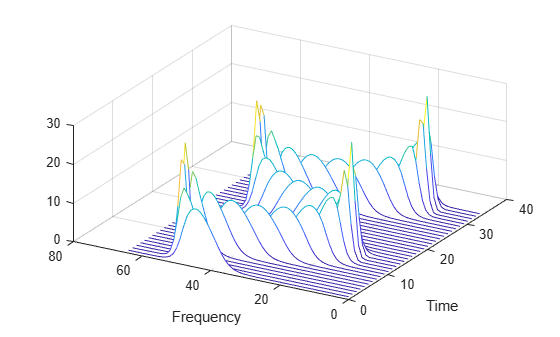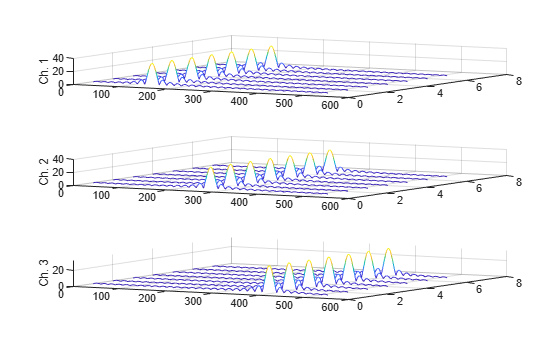# stftLayer

Short-time Fourier transform layer

## Description

An STFT layer computes the short-time Fourier transform of the input. Use of this layer requires Deep Learning Toolbox™.

## Creation

### Syntax

``layer = stftLayer``
``layer = stftLayer(Name=Value)``

### Description

example

````layer = stftLayer` creates a Short-Time Fourier Transform (STFT) layer. The input to `stftLayer` must be a `dlarray` (Deep Learning Toolbox) object in `"CBT"` format with a size along the time dimension greater than the length of `Window`. `stftLayer` formats the output as `"SCBT"`. For more information, see Layer Output Format. NoteThe weights in `stftLayer` are initialized internally to be the modulated windows used as filters in the STFT. It is not recommended to initialize the weights directly. ```

example

````layer = stftLayer(Name=Value)` sets properties using one or more name-value arguments. You can specify the analysis window and the number of overlapped samples, among others.```

## Properties

expand all

### STFT

Analysis window used to compute the STFT, specified as a vector with two or more elements.

Example: `(1-cos(2*pi*(0:127)'/127))/2` and `hann(128)` both specify a Hann window of length 128.

Data Types: `double` | `single`

Number of overlapped samples, specified as a positive integer strictly smaller than the length of `Window`.

The stride between consecutive windows is the difference between the window length and the number of overlapped samples.

Data Types: `double` | `single`

Number of frequency points used to compute the discrete Fourier transform, specified as a positive integer greater than or equal to the window length. If not specified, this argument defaults to the length of the window.

If the length of the input data along the time dimension is less than the number of DFT points, `stftLayer` right-pads the data and the window with zeros so they have a length equal to `FFTLength`.

Data Types: `double` | `single`

Layer transform mode, specified as one of these:

• `"mag"` — STFT magnitude

• `"squaremag"` — STFT squared magnitude

• `"logmag"` — Natural logarithm of the STFT magnitude

• `"logsquaremag"` — Natural logarithm of the STFT squared magnitude

• `"realimag"` — Real and imaginary parts of the STFT, concatenated along the channel dimension

Data Types: `char` | `string`

### Layer

Multiplier for weight learning rate, specified as a nonnegative scalar. If not specified, this property defaults to zero, resulting in weights that do not update with training. You can also set this property using the `setLearnRateFactor` (Deep Learning Toolbox) function.

Data Types: `double` | `single`

Layer name, specified as a character vector or a string scalar. For `Layer` array input, the `trainNetwork` (Deep Learning Toolbox), `assembleNetwork` (Deep Learning Toolbox), `layerGraph` (Deep Learning Toolbox), and `dlnetwork` (Deep Learning Toolbox) functions automatically assign names to layers with the name `''`.

Data Types: `char` | `string`

Number of inputs of the layer. This layer accepts a single input only.

Data Types: `double`

Input names of the layer. This layer accepts a single input only.

Data Types: `cell`

Number of outputs of the layer. This layer has a single output only.

Data Types: `double`

Output names of the layer. This layer has a single output only.

Data Types: `cell`

## Examples

collapse all

Generate a signal sampled at 600 Hz for 2 seconds. The signal consists of a chirp with sinusoidally varying frequency content. Store the signal in a deep learning array with `"CTB"` format.

```fs = 6e2; x = vco(sin(2*pi*(0:1/fs:2)),[0.1 0.4]*fs,fs); dlx = dlarray(x,"CTB");```

Create a short-time Fourier transform layer with default properties. Create a `dlnetwork` object consisting of a sequence input layer and the short-time Fourier transform layer. Specify a minimum sequence length of 128 samples. Run the signal through the `predict` method of the network.

```ftl = stftLayer; dlnet = dlnetwork([sequenceInputLayer(1,MinLength=128) ftl]); netout = predict(dlnet,dlx);```

Convert the network output to a numeric array. Use the `squeeze` function to remove the length-1 channel and batch dimensions. Plot the magnitude of the STFT. The first dimension of the array corresponds to frequency and the second to time.

```q = extractdata(netout); waterfall(squeeze(q)') set(gca,XDir="reverse",View=[30 45]) xlabel("Frequency") ylabel("Time")```Generate a 3 × 160 (× 1) array containing one batch of a three-channel, 160-sample sinusoidal signal. The normalized sinusoid frequencies are π/4 rad/sample, π/2 rad/sample, and 3π/4 rad/sample. Save the signal as a `dlarray`, specifying the dimensions in order. `dlarray` permutes the array dimensions to the `"CBT"` shape expected by a deep learning network.

```nch = 3; N = 160; x = dlarray(cos(pi.*(1:nch)'/4*(0:N-1)),"CTB");```

Create a short-time Fourier transform layer that can be used with the sinusoid. Specify a 64-sample rectangular window, 48 samples of overlap between adjoining windows, and 1024 DFT points. By default, the layer outputs the magnitude of the STFT.

```stfl = stftLayer(Window=rectwin(64), ... OverlapLength=48, ... FFTLength=1024);```

Create a two-layer `dlnetwork` object containing a sequence input layer and the STFT layer you just created. Treat each channel of the sinusoid as a feature. Specify the signal length as the minimum sequence length for the input layer.

```layers = [sequenceInputLayer(nch,MinLength=N) stfl]; dlnet = dlnetwork(layers);```

Run the sinusoid through the `forward` method of the network.

`dataout = forward(dlnet,x);`

Convert the network output to a numeric array. Use the `squeeze` function to collapse the size-1 batch dimension. Permute the channel and time dimensions so that each array page contains a two-dimensional spectrogram. Plot the STFT magnitude separately for each channel in a waterfall plot.

```q = squeeze(extractdata(dataout)); q = permute(q,[1 3 2]); for kj = 1:nch subplot(nch,1,kj) waterfall(q(:,:,kj)') view(30,45) zlabel("Ch. "+string(kj)) end```expand all

## Version History

Introduced in R2021b

expand all